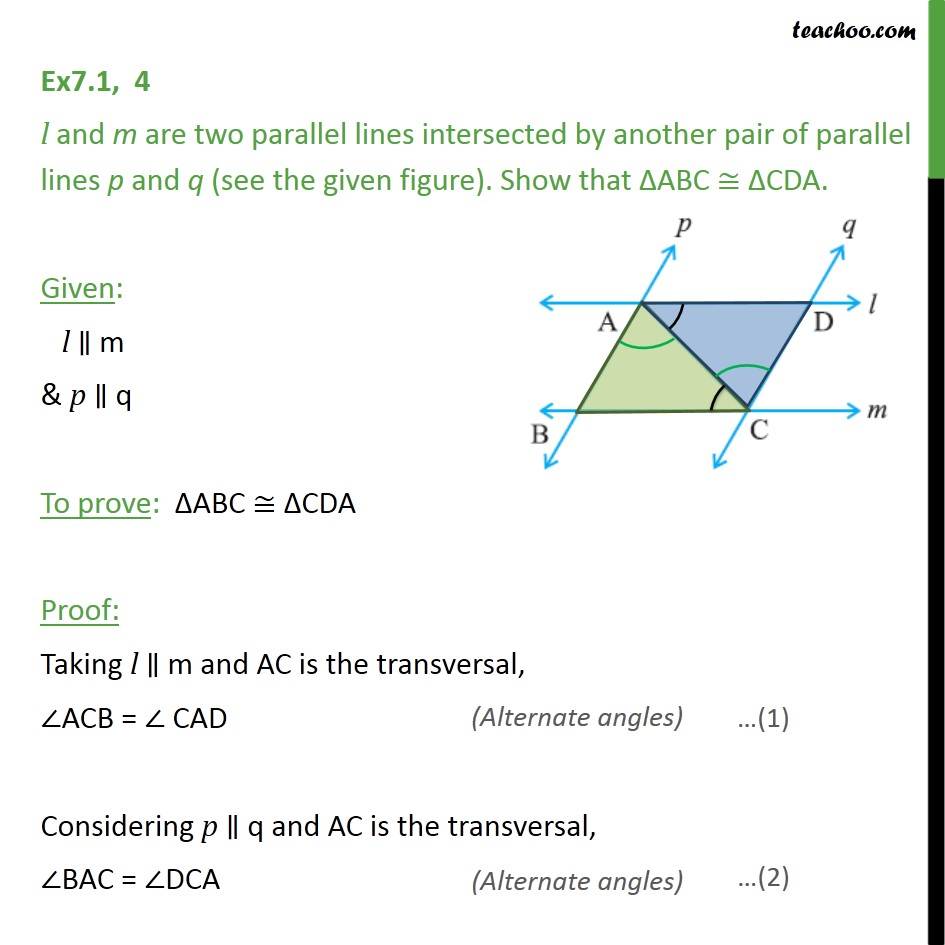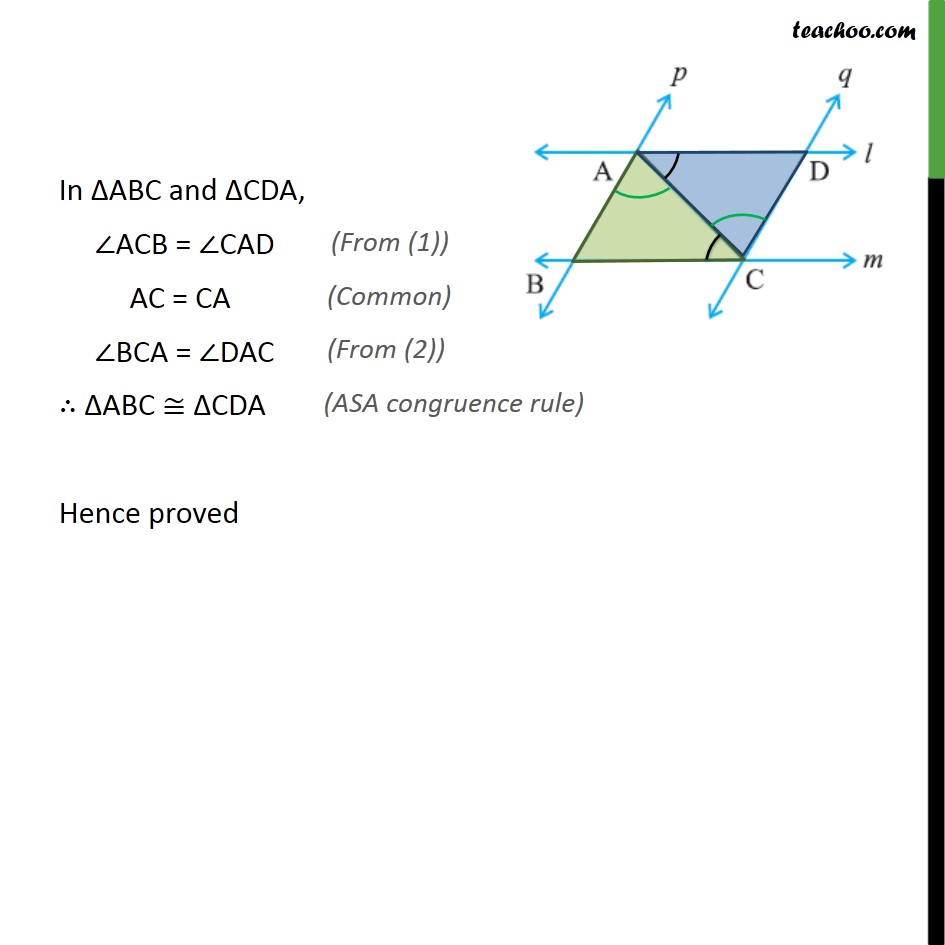1. Chapter 7 Class 9 Triangles (Term 1)
2. Serial order wise
3. Ex 7.1

Transcript

Ex7.1, 4 l and m are two parallel lines intersected by another pair of parallel lines p and q (see the given figure). Show that ABC CDA. Given: l m & p q To prove: ABC CDA Proof: Taking l m and AC is the transversal, ACB = CAD Considering p q and AC is the transversal, BAC = DCA In ABC and CDA, ACB = CAD AC = CA BCA = DAC ABC CDA Hence proved

Ex 7.1